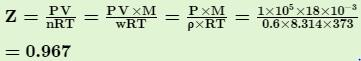Courses

# Test: Van Der Waals Equation

## 21 Questions MCQ Test Chemistry for JEE | Test: Van Der Waals Equation

Description
This mock test of Test: Van Der Waals Equation for JEE helps you for every JEE entrance exam. This contains 21 Multiple Choice Questions for JEE Test: Van Der Waals Equation (mcq) to study with solutions a complete question bank. The solved questions answers in this Test: Van Der Waals Equation quiz give you a good mix of easy questions and tough questions. JEE students definitely take this Test: Van Der Waals Equation exercise for a better result in the exam. You can find other Test: Van Der Waals Equation extra questions, long questions & short questions for JEE on EduRev as well by searching above.
QUESTION: 1

### Direction (Q. Nos. 1-11) This section contains 11 multiple choice questions. Each question has four choices (a), (b), (c) and (d), out of which ONLY ONE option is correct. Q. In van der Waals’ equation of state for non-ideal gas, that includes correction for intermolecular forces is [ITT JEE 1998, 2009]

Solution:

For correction in intermolecular forces, we have ∆P = an2/V2
So the actual pressure becomes, P+an2/V2

QUESTION: 2

### The value of van der Waals’ constant a for various gases are given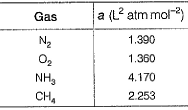Q. The order of liquefaction of these gases is [IITJEE 1989]

Solution:

More the value of a, more be easy to liquify the gas.
So, the order of ease of liquefaction is, NH3 > CH4 > N2 > O2

QUESTION: 3

### A gas will approach ideal behavious at  [IIT JEE 1999]

Solution:

At high temperature and low pressure, the gas volume is infinitely large and both intermolecular force as well as molecular volume can be ignored. Under this condition postulates of kinetic theory applies appropriately and gas approaches ideal behavior.

QUESTION: 4

The compressibility of a gas is less than unity for a gas at STP. Therefore,

[IIT JEE 2000]

Solution:
QUESTION: 5

Positive deviation from ideal behaviour takes place because of

[IIT JEE 2003]

Solution:

Compressibility factor of an ideal gas :

z = pV/nRT = 1

If a gas is deviated from its ideal behaviour, therefore, z >1 or 1 > z.

Hence, for positive deviation of a gas from ideal behaviour  z > 1.

QUESTION: 6

Van der Waals ’ equation for one mole of CO2 gas at low pressure will be

Solution:

At low pressure, volume correction for 1 mole of gas is negligible,
b=0 and van der Waals equation becomes
[P+a/V2]V=RT
So, the correct option is A

QUESTION: 7

If V is the volume of one molecule of a gas under given conditions, then van der Waals ’ constant b (also called excluded volume or effective volume) is

Solution:

`b` is equal to 4 times the volume of molecules in one mole of a gas N0 molecules
Volumes of one molecule = v
Volume of N0 molecule =vN0
Hence, b=4vN0

QUESTION: 8

Fraction of the effective volume occupied by molecules in 1 mole of a gas at STP (diameter of the molecule is 2 x 10-10m) is

Solution:
QUESTION: 9

The compressibility factors for real gases at low pressure , high pressure and that of gases of low molar masses are Z1 Z2 and Z3. These are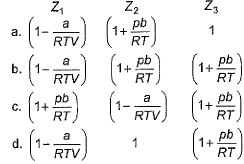[AIEEE 2012 , JEE Main 2014]

Solution:

The compressibility factor for real gases at low pressure, high pressure and that of gases of low molar masses are z1, z2 and z3.The values of z1, z2, z3 can be derived as follows.
At low pressures, the gas equation can be written as,
(P + a/v2m) (Vm) = RT
or Z1 = Vm / RT = 1 – a/VmRT
At higher pressure,
PV - Pb = RT
So, equating with real gas equation,
PV / RT = 1 + Pb/RT
Again, Z2 = PV / RT
So, Z2 = 1 + Pb / RT

QUESTION: 10

a and b are van der Waals’ constants for gases. Chlorine is more easily liquefied than ethane because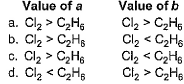[AIEEE 2011]

Solution:

Van der waals constant ′a′ is a mixture of inter molecular forces and ′b′ is a measure of molecular size. A greater value of ′a′ and lesser value of ′b′ supports qualification of a given gas. Therefore, chlorine is more easily liquefied than ethane because
′a′ for Cl2 > ′a′ for C2H6 but ′b′ for Cl2 > ′b′ for C2H6.

QUESTION: 11

For one mole of a van der Waals’ gas when b = 0 and T = 300 K, the pV versus 1/V plot is shown in figure. The value of the van der Waals' constant a (atm L2 mol-2) is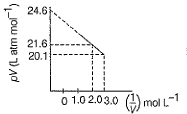Solution:

The correct answer is option B
Vanderwaal's equation for 1 mole of gas is
(P + a/V2 ​)( V − b ) = RT
But, b = 0......(given)
(P + a/V2​) (V) = RT
∴PV= (−a × 1/V​) + RT
We know the basic general equation of straight line, y= mx + c
Slope = tan( π − θ ) = −tan θ = −a
So, tanθ = a =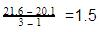QUESTION: 12

Direction (Q. Nos. 12) Choice the correct combination of elements and column I and coloumn II  are given as option (a), (b), (c) and (d), out of which ONE option is correct.

Q. Match the parameters given in Column I with the units given in Column II and select the correct answer from the codes given below.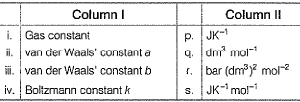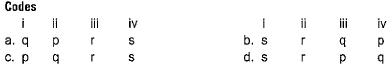Solution:

‘R’ = J mol-1K-1
‘a’ = atm L2 mol-2 = bar (dm3)2 mol-2
‘b’ =  L mol-1 = dm3 mol-1

*Multiple options can be correct
QUESTION: 13

Direction (Q. Nos. 13-15) This section contains 3 multiple choice questions. Each question has four choices (a), (b), (c) and (d), out of which ONE or  MORE THANT ONE  is correct.

Q. The given graph represent the variation of Z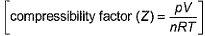versus p, for three real gases A, B and C. Select correct statement(s).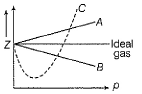Solution:

According to a real gas equation,
The constants 'a'  and 'b': Van der Waals constant for attraction 'a' and volume 'b' are characteristic constants for a given gas.
(i)The 'a' values for a given gas are a measure of intermolecular forces of attraction. More are the intermolecular forces of attraction, more will be the value of a.
(ii)For a given gas van der Waals constant of attraction 'a' is always greater than van der Waals constant of volume 'b'.
(iii)The gas having a higher value of 'a'  can be liquefied easily and therefore H2 and He is not liquefied easily.
According to this, for gas A (Z>1), a=0 and its dependence on P is linear at all pressure and for gas B (Z<1), b=0 and its dependence on P are linear at all pressure.
Also, at high pressure, the slope is positive for all real gases.

*Multiple options can be correct
QUESTION: 14

When two gaseous molecules of equal radius r collide,

Solution: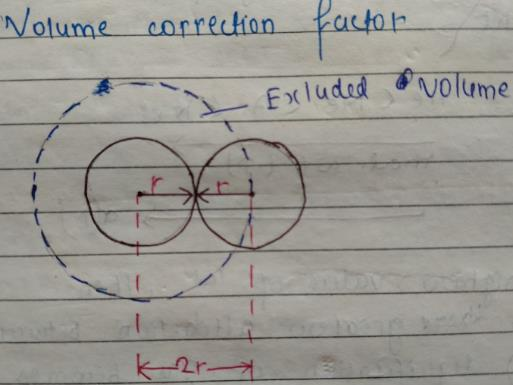From the figure, their centres are separated by 2r at the point of contact
Volume of 1 molecule = 4/3 πr3 = Vmol
Effective volume = 4/3 π(2r)3 = 8 4/3πr3 = 8Vmol
Excluded volume per molecule = ½ 4/3 π(2r)3 = ½ 8 4/3πr3 = 4Vmol
Greater the excluded volume, greater the deviation from ideal behaviour.

*Multiple options can be correct
QUESTION: 15

Select the correct statements based on the virial equation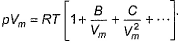.

The coefficient B in the equation of state

Solution:

The coefficient B in the virial equation on state PVm=RT(1+B/Vm+ C/Vm2+...)
b.is equal to zero at Boyle’s temperature. At this temperature, the real gas behaves like ideal gas and the virial equation becomes ideal gas equation  PVm=RT
c. has the dimension of molar volume. This is because in the virial equation, it appears in the form of the ratio B/Vm
d. B = b - a/RT is the relation b/w B aand van er walls constant.

QUESTION: 16

Direction (Q. Nos. 16 and 17) This sectionis based on statement I and Statement II. Select the correct answer from the code given below.

Q.

Statement I : At constant temperature, pV versus p isotherm for real gases is not a straight line.

Statement II : At high pressure, all gases have Z > 1, but at intermediate pressure, most gases have Z < 1.

Solution:

Assertion is true. For real gas, pV vs p plot is not a straight line.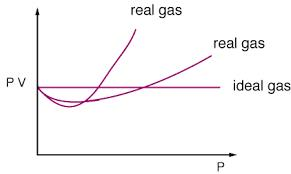The reason for this is that for real gas, we have to consider the intermolecular forces which are ignored in ideal gas.
Reason is also correct.Z>1at higher  pressure for all gas but at low pressure, some gases have Z<1. However this is not the correct explanation of assertion.

QUESTION: 17

Statement I : In van der Waals’ equation, pressure correction is due to force of attraction between molecules.

Statement II : Rate of change of momentum is equal to force.

Solution:

Vander waals constant 'a' is a measure of attractive force
The van der waals constant 'a' represents the magnitude of the attractive forces present between gas molecules. Higher is the value of 'a', more easily the gas can be liquefied.
However it has no relation with statement II. Statement II is a general statement.

QUESTION: 18

Direction (Q. Nos. 18-20) This section contains a paragraph, wach describing theory, experiments, data etc. three Questions related to paragraph have been given.Each question have only one correct answer among the four given options (a),(b),(c),(d).

For CO, variation of pV with p at constant temperature is given by isotherm shown in figure.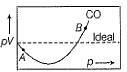Q. Near the point A, compressibility factor Z is

Solution:

Compressibility factor(Z) = PV/RT
For 1 mole of real gas, Van der Waals eqn is (P + a/V2)(V-b) = RT
At low pressure, volume is very large and hence correction term; b can be neglected in comparison to V
(P + a/V2)V= RT
PV + a/V = RT
PV/RT = 1- a/VRT
Z = 1-a/VRT

QUESTION: 19

For CO, variation of pV with p at constant temperature is given by isotherm shown in figure.Q. Near the point B, Compresibility factor Z is

Solution:

Above Z=1, we have Z>1 and below Z=1, we have Z<1. So, the correct answer be (1+b/V)

QUESTION: 20

For CO, variation of pV with p at constant temperature is given by isotherm shown in figure.Q. For which gas. Z has the same relation as at point B

Solution:

For H2, we have always Z > 1. In the given graph, at position B we have Z > 1.

*Answer can only contain numeric values
QUESTION: 21

Direction (Q. Nos. 21) This section contains 1 questions. when worked out will result in an integer from 0 to 9 (both inclusive).

Q. The density of steam at 100.0°C and 1.0x 105 Pa is 0.6 kg m-3. Thus, compressibility factor (Z) deviates from the normal value under ideal condition is ...... %.

Solution:

As we know, comprssibility factor (Z)